## Curriculum Aligned K-6 Online Maths Worksheets and Activites

PARENTS TEACHERS•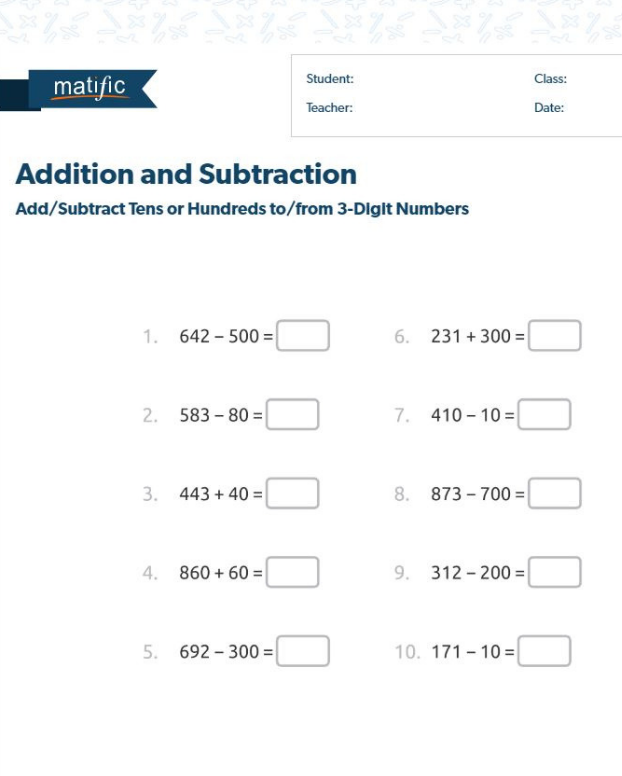Counting

This worksheet helps practise adding & subtracting tens or hundreds to and from 3 digit numbers.

•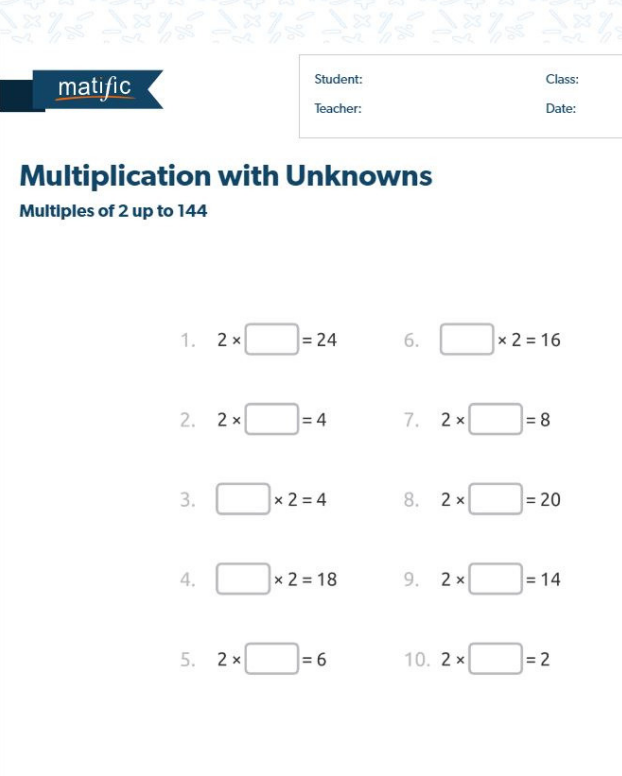Multiplication

#### Multiplication with Unknowns

This worksheet helps students develop strategies for memorising multiplication facts.

•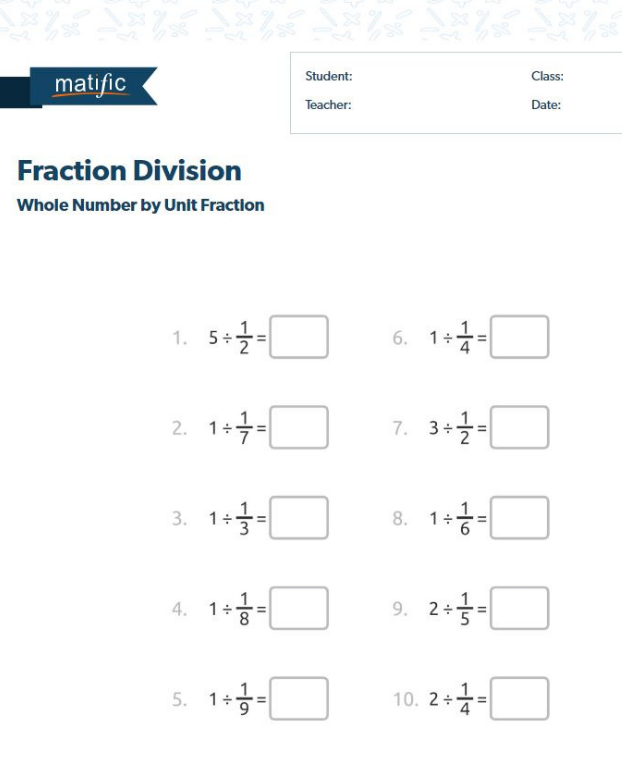Fractions

#### Fraction Division

This worksheet helps students practise dividing a whole number by a unit fraction.

### Examples of Digital Maths Worksheets

•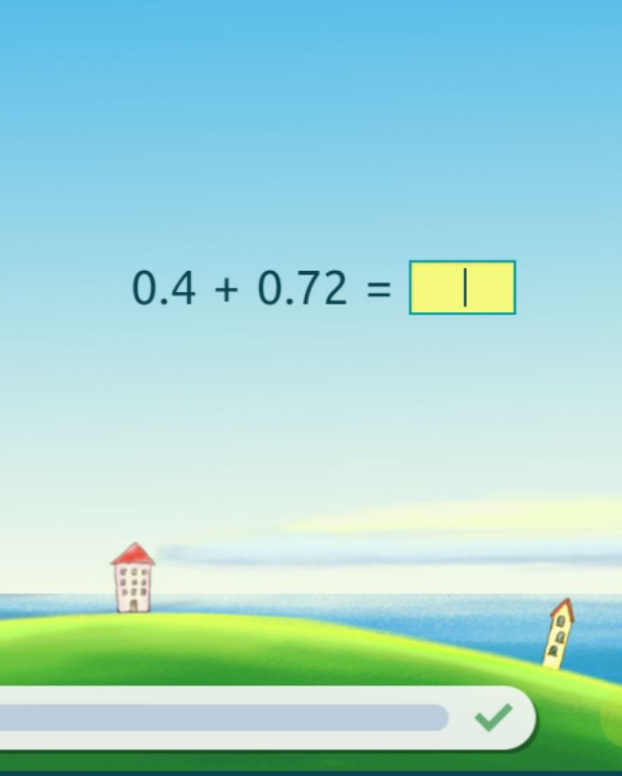Decimals

This digital worksheet helps students learn how to add and subtract up to two decimal places.

•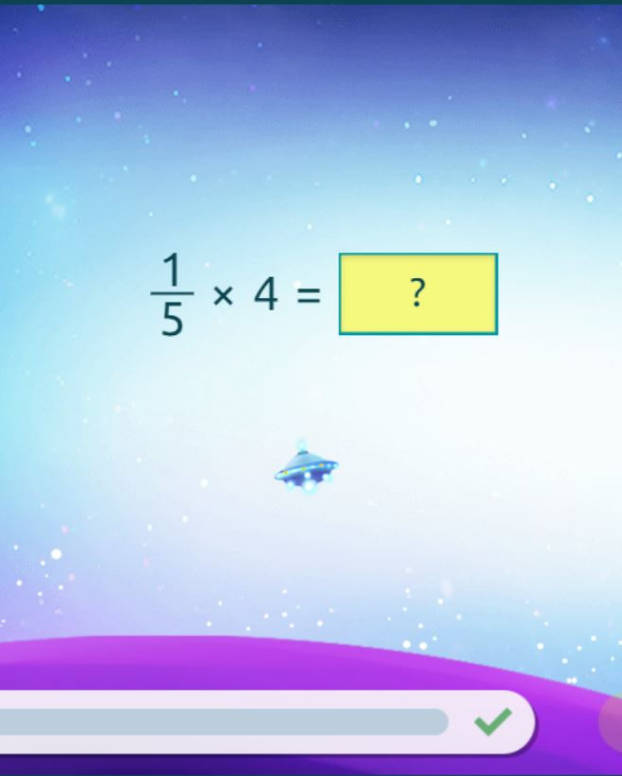Fractions

#### Fraction Multiplication

This digital worksheet helps students practise multiplying proper and improper fraction.

•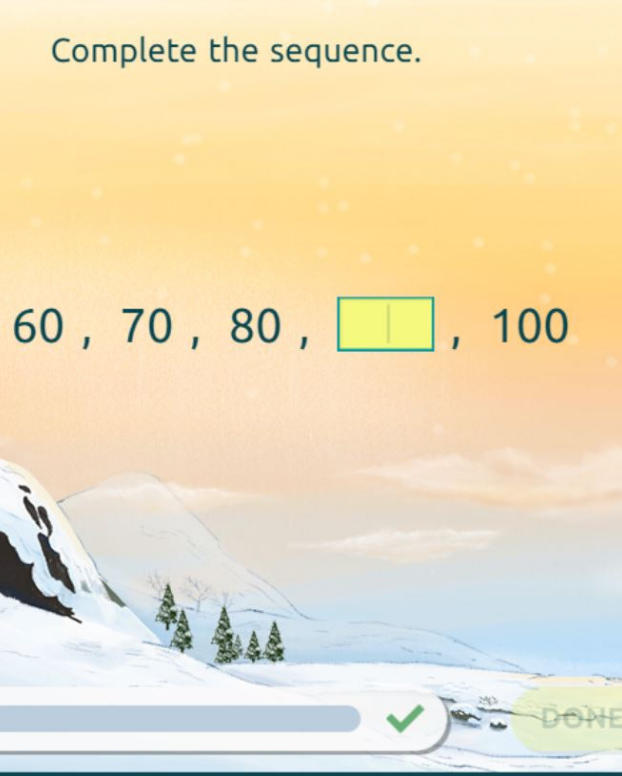Sequence

#### Arithmetic Progressions

This digital worksheet helps students practise arithmetic sequences for multiplies of ten.

### Examples of Online Maths Activities

•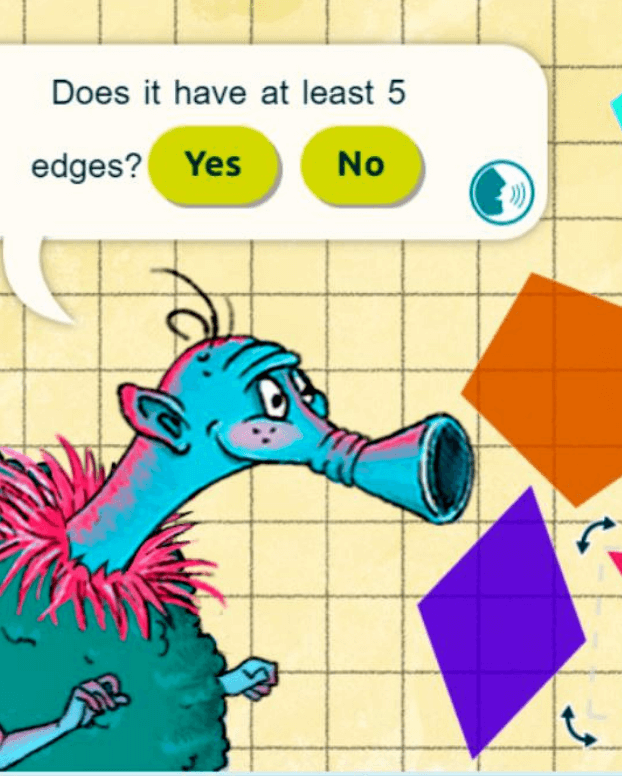Geometry

#### Identifying Polygons

This activity helps students practise the classification of polygons according to the number of edges or the number of vertices.

•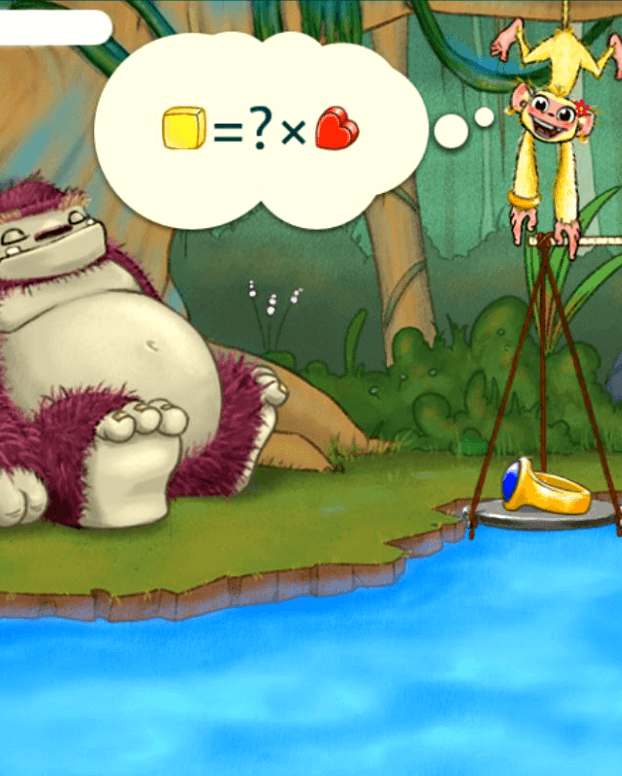Algebra

#### Reasoning & Problem-Solving

This activity involves finding unknown quantities in number sentences using addition and subtraction.

•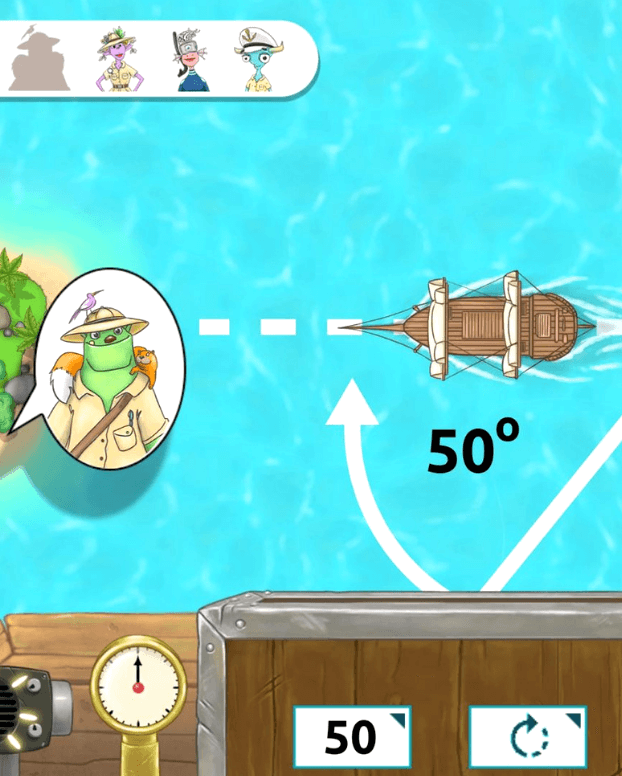Measurements

#### Measuring Angles

This activity helps students measure angles using a digital protractor in a real-life setting.

## Browse and Assign Curriculum Aligned Maths Worksheets and Activities

•Sign up now to browse curriculum aligned online maths activities, worksheets, lesson plans and more!

•Assign activities for addition, subtraction, multiplications, fractions, geometry, word problems, and other maths lessons.

•Give your students direct access from your Matific account to start completing interactive, hands-on maths activities and games.

## Suggested Ways to Use Matific in the ClassroomAssign Work Choose the most relevant work for your students, and assign this work to your students. Track Progress Track your students completion and performance with our real time and automated reports.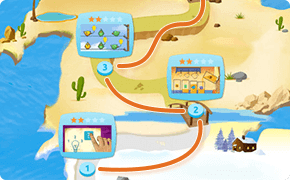Each student will receive a digital path way, which contains all the activities for their year level.Students can simply log in, and complete the work in their BONUS path at their own pace, as well as any specific work you have chosen for them.Smart Algorithm If you like the idea of AutoAssign, Matific is smart enough to know which work to give to your class each week. Autoassign As your class completes work, our algorithm will automatically adjust to Auto Assign the most relevant activities to your classes.

## Awards of excellence in education

••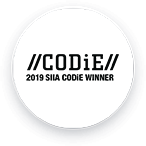•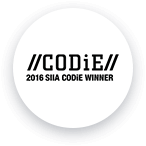•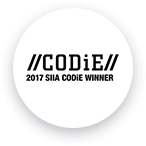••••Matific v4.1.0